[Top] [Next] [Prev]

B.4 MOS Transistors

We now turn our attention to logic functions constructed from MOS transistors, the dominant technology of today.

B.4.1 Voltage-Controlled Switches

The operation of an MOS transistor is considerably easier to explain than that of a bipolar transistor. The voltage-controlled switches introduced in Section 1.3.1 correspond directly to MOS transistors. In this section, we describe the operation of MOS transistors and how they can be used to implement logic gates.

An MOS transistor is nothing more than a voltage-controlled switch. It has three connection points: a source, a drain, and a gate (a transistor gate bears no resemblance to a logic gate, an unfortunately ambiguous use of the term).

A cross section of the metal-oxide-silicon sandwich that forms the transistor is shown in Figure B.12.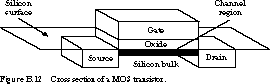The bottommost material layer is made of silicon, an insulating oxide layer sits on top of it, and the topmost layer is the metal gate. (More modern integrated circuit processes have replaced the metal layer with a material called polycrystalline silicon, but the older "metal gate" terminology still holds.) The source and drain regions contain silicon material with a large excess of electrons separated by the slightly positively charged bulk silicon. The source and drain are called diffusion regions because of the chemical process used to create them. Negatively charged ions (atoms with extra valence electrons) are placed onto the silicon surface and are diffused into the surface by heating the silicon material. The materials of the source and drain are identical. By convention, the source is the electrical node with the lower of the two voltage potentials at either end of the channel.

The electrical behavior of the transistor is generally as follows. When a positive voltage is placed on the gate, electrons from the silicon bulk are attracted to the transistor channel, an initially nonconducting region between the source and drain very close to the silicon surface. When the gate voltage becomes sufficiently positively charged, enough electrons are pulled into the channel from the bulk to establish a charged path between the source and the drain. Electrons flow across the transistor channel, and the voltage-controlled switch is conducting. If a 0 or very small voltage is placed on the gate, no electrons (or at least very few) are attracted to the channel. The source and drain are disconnected, no current flows across the channel, and the switch is not conducting.

There are two fundamentally different kinds of MOS transistors, called n-channel and p-channel transistors, or nMOS and pMOS for short. Their schematic symbols are shown in Figure B.13.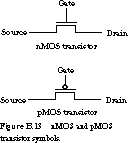Because they are made from materials with different affinities for electrons, the two transistor types behave quite differently. The transistor operation described above is actually for the nMOS transistor. The bulk is positively charged, while the diffusion is negatively charged. The transistor switch is "closed" (conducting) when a logic 1 is placed on its gate and "open" (nonconducting) when the gate is connected to a logic 0. The pMOS transistor is complementary. The diffusion regions are positively charged and the silicon bulk is negatively charged. A pMOS transistor behaves in a complementary way: It is "closed" (conducting) when a logic 0 is placed on the gate and is "open" (nonconducting) when a logic 1 is placed there.

The symbols for the two different kinds of transistors make it easy to remember how they operate. An nMOS transistor conducts when the gate voltage is asserted in positive logic. The pMOS transistor conducts when the gate is asserted in negative logic. This is why there is a polarity bubble on the gate of the pMOS transistor's symbol.

B.4.2 Logic Gates from MOS Switches

Any logic gate can be constructed from a combination of nMOS and pMOS transistors.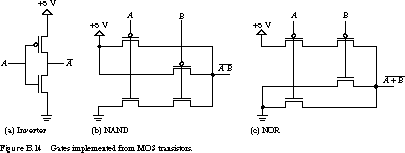Figure B.14 shows transistor networks for (a) an inverter, (b) a two-input NAND gate, and (c) a two-input NOR gate. The power supply (+5 V) and ground (0 V) represent logic 1 and logic 0, respectively. The inverter is constructed from an nMOS and a pMOS transistor connected in series between power and ground. It operates as follows (see Figure B.15).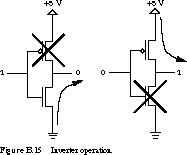When A is a logic 1, the nMOS transistor is conducting and the pMOS transistor is not. The only unbroken connection path is from ground to the output node. Thus, a logic 1 at the input yields a logic 0 at the output.

Now let's look at the case in which A is a logic 0. Now the pMOS transistor conducts while the nMOS transistor does not. The output node is connected to a logic 1. A 0 at the input yielded a 1 at the output. The series transistors implement an inverter.

The transistor-level implementations of the NAND and NOR gate work similarly. Let's start with the NAND logic gate, constructed from two nMOS transistors in series between the output node and ground and two pMOS transistors in parallel between the output node and the power supply. A path between the output node and ground can be established only when both of the nMOS transistors are conducting. This happens only if A and B are both at a logic 1. In this case, the two pMOS transistors are not conducting, breaking all paths between the output node and the logic 1 at the power supply. This is the case A = B = 1, output = 0.

Now what happens if one or both of A and B are at a logic 0? Let's take the case A = 0 and B = 1 (see Figure B.16).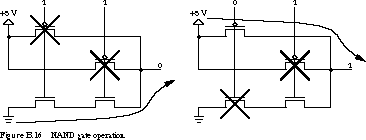The nMOS transistor controlled by A is not conducting, breaking the path from the output to ground. The pMOS transistor it controls is conducting, establishing the path from the power supply to the output. The other path, controlled by B, is broken, but this has no effect on the output node as long as some path exists between it and some voltage source.

The case A = 1 and B = 0 is symmetric. If both inputs are 0, there are now two paths between the power supply's logic 1 and the output node. Of course, this doesn't change the logic signal at the output: it is still a logic 1. From this discussion it should be obvious that the circuit configuration performs the function of a NAND gate.

The pull-down network switches between the output and the ground signal. We define the pull-up network similarly between the output and the power supply. The pull-down network is A B, since the transistors are two nMOS transistors in series. The pull-up network consists of two pMOS devices in parallel. This logic function is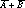. Another way to say this is that the function is 0 when A B is true and is 1 whenis true. Of course, by DeMorgan's theorem,is the same function as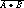.

We can apply the same kind of analysis to the NOR gate implemented as transistors. Using the observation we just made, the pull-up network is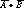, the pull-down network is A + B, and the function is 0 when A + B is true and is 1 when(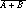) is true. Analyzing the transistor network directly (see Figure B.17), the output node can be 1 only if both transistors between it and the power supply are conducting. Thus the output is 1 if both inputs are 0. If either or both inputs are 1, then the path to the power supply is broken while at least one path from the output to ground is established. The network does indeed implement the NOR function.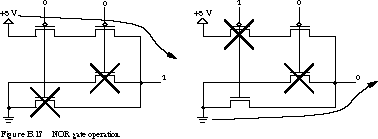B.4.3 CMOS Transmission Gate

Any gate logic function can be implemented as a pull-up network of pMOS transistors and a pull-down network of nMOS transistors. In fact, this is the standard way to construct digital logic from CMOS transistors. Yet there is an even richer set of things you can do with switching networks. The key to constructing these networks is a special circuit structure called the transmission gate. We examine it next.

For electrical reasons that are beyond the scope of this discussion, it turns out that pMOS transistors are great at transmitting a logic 1 voltage without signal loss, but the same cannot be said about logic 0 voltages. Having 0 V at one side of a conducting pMOS transistor yields a voltage at the other side somewhat higher than 0 V. NMOS transistors have a -comp-lementary problem: they are great at passing logic 0 but awful at passing logic 1. In the circuits we have looked at so far, pMOS transistors in the pull-up network passed only ones while the nMOS transistors in the pull-down network passed only zeros. So everything works out fine.

As you may guess, the best possible transmission behavior can be -obtained by combining both kinds of transistors. This yields the CMOS transmission gate, which is shown in Figure B.18.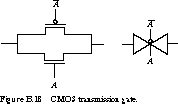The pMOS and nMOS transistors are connected in parallel and are controlled by complementary control signals in the figure. When signal A is asserted, the transmission gate conducts a logic 0 or 1 equally well. Signal A at a logic 1 makes the nMOS transistor conduct, while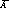at a logic 0 makes the parallel pMOS transistor conduct as well. When A is unasserted, the gate no longer conducts. A at logic 0 breaks the connection through the nMOS transistor, whileat 1 has the same effect on the pMOS transistor. In circuit diagrams, the transmission gate is often denoted by a "butterfly" or "bow tie" symbol, as shown in the figure.

Section 4.2 covers how to use CMOS transmission gates to implement digital subsystems with many fewer transistors than would be the case if traditional gates were used.

[Top] [Next] [Prev]